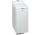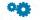# Hands

The clock shows 12 hours. After how many minutes will angle between the hour and minute hand 90°?

Consider the continuous movement of both hands hours.

Result

x =  16.36 min

#### Solution:

360/60*x - 360/(12*60)*x = 90

330x = 5400

x = 18011 ≈ 16.363636

Calculated by our simple equation calculator.

Our examples were largely sent or created by pupils and students themselves. Therefore, we would be pleased if you could send us any errors you found, spelling mistakes, or rephasing the example. Thank you!

Leave us a comment of this math problem and its solution (i.e. if it is still somewhat unclear...):Be the first to comment!#### Following knowledge from mathematics are needed to solve this word math problem:

Need help calculate sum, simplify or multiply fractions? Try our fraction calculator. Do you have a linear equation or system of equations and looking for its solution? Or do you have quadratic equation? Do you want to convert length units?

## Next similar math problems:

1. ClocksHow long path will walk the end of the clock's minute-hand long 7 cm for 330 minutes? How long will walk in the same time hour-hand?
2. Bicycle wheelA bicycle wheel has a diameter of 60 cm. Approximately how many times does wheel rotate at 2.5 km long trip?
3. PizzaPizza with a diameter 50 cm have weight 559 g. What diameter will have a pizza weighing 855 g if it is make from the same cloth (same thickness....) and same decorated?
4. Circle arcCircle segment has a circumference of 135.26 dm and 2096.58 dm2 area. Calculate the radius of the circle and size of central angle.
5. Earth parallelEarth's radius is 6370 km long. Calculate the length parallel of latitude 50°.
6. ArcCircle arc corresponding to angle is 32° is 28 dm long. What is the length of the entire circle?
7. Quarter circularThe wire that is hooked around the perimeter of quarter-circular arc has length 3π+12. Determine the radius of circle arc.
8. RectangleThe rectangle is 21 cm long and 38 cm wide. Determine the radius of the circle circumscribing rectangle.
9. WasherWashing machine drum wash at 58 RPM. Washing machine motor pulley has diameter 9 cm. What must be the diameter of the drum machine pulley when the motor is at 438 RPM?
10. CylindersArea of the side of two cylinders is same rectangle of 33 mm × 18 mm. Which cylinder has a larger volume and by how much?
11. GearTwo gears, fit into each other, has transfer 2:3. Centres of gears are spaced 82 cm. What are the radii of the gears?
12. ConeCircular cone of height 15 cm and volume 5699 cm3 is at one-third of the height (measured from the bottom) cut by a plane parallel to the base. Calculate the radius and circumference of the circular cut.
13. CircleHow big is area of circle if its circumference is 80.6 cm?
14. Cu wireCopper wire has a length l = 980 m and diameter d = 8 mm. Calculate the weight if density of copper is ρ = 8500 kg/m3. Result round to one decimal place.Determine the radius of the circle, if its perimeter and area is the same number.Calculate the dimensions of rotating cylindrical container with volume 2 l, if height of container is equal to the diameter of the base.Calculate the radius (circumradius) of the circle described right triangle with hypotenuse long 33 and one cathetus long 17.